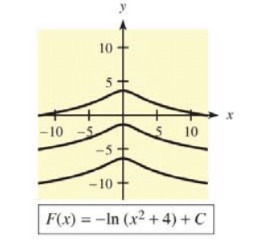Chapter 12.3, Problem 38E### Mathematical Applications for the ...

11th Edition
Ronald J. Harshbarger + 1 other
ISBN: 9781305108042

#### Solutions

Chapter
Section### Mathematical Applications for the ...

11th Edition
Ronald J. Harshbarger + 1 other
ISBN: 9781305108042
Textbook Problem

# In Problems 37-40, a family of functions is given and graphs of some members are shown. Find the function f ( x ) such that the family is given by ∫ f ( x ) d x . F ( x ) = − ln ( x 2 + 4 ) + CTo determine

The function f(x) such that the family is given by f(x)dx=F(x) where F(x)=ln(x2+4)+C

Explanation

Given information:

The provided graph is:

The provided function is:

F(x)=ln(x2+4)+C

Explanation:

Consider the provided graph of the function:

And the provided functions is:

F(x)=x+ln|x|+C

If F'(x)=f(x)

Now find out the first derivative of the provided function as:

f(x

### Still sussing out bartleby?

Check out a sample textbook solution.

See a sample solution

#### The Solution to Your Study Problems

Bartleby provides explanations to thousands of textbook problems written by our experts, many with advanced degrees!

Get Started

#### Find more solutions based on key concepts